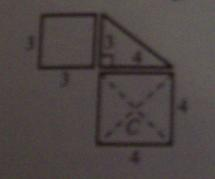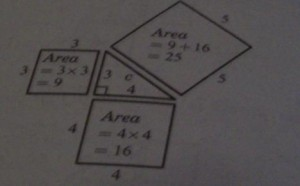+639178252242 [email protected]

For my 2nd post in the category educational aid, I will tackle another subject, and this time it’s Trigonometry, or which other students know as Mathematics. I will try to post more and more lessons within a single day soon, but let’s keep it for 1 lesson a day for now.

### The Introduction

Of all geometric figures, the right triangle is perhaps the most important. The subject of trigonometry, or “triangle measurement,” began with observations about the properties of similar right triangles. These observations led to the creation of the trigonometric rations, three of which are sine, cosine, and tangent. These ratios have turned out to be useful in working with triangles, other than right triangles, and even in problems that have no connection with triangles at all.

### The Pythagorean Theorem

Pythagoras was a Greek mathematician who lived at about 540 BC. He is best known for his theorem concerning right triangles. Although, the Babylonians had discovered this theorem much earlier, Pythagoras was recognized as the first to prove it. The Chinese were also familiar with the theorem at about the same time as Pythagoras.

### Before we discuss the theorm, let us study the following:

A triangle with legs of length, say, 3 units and 4 units is drawn.Squares are then drawn against the two legs.

Note that C is the center of the larger square.Segment AB is drawn parallel to the hypotenuse. Segment EF is drawn perpendicular to the hypotenuse. Both Segment AB and Segment EF should pass through C.The larger square is then cut into four pieces, along segment AB and segment EFObserver how the five pieces are arranged to form a square along the hypotenuse.

The same result can be shown for a right riangle of any size. Furthermore, this procedure enables us to find the length of the hypotenuse of the triangle given in Figure 1.4

Let the length of the hypotenuse be c. Thus, the area of the square on the hypotenuse will be equal to c x c = c2

Now, the area of the square on the hypotenuse will be equal to: 9+16=25 (Figure 1.5)
For example, if c2=25, then c=√25=5Therefore, a right triangle with legs of 3 units and 4 units long, has a hypotenuse of 5 units long.

```And this has been Lesson Number 1 for 4th Year Trigonometry